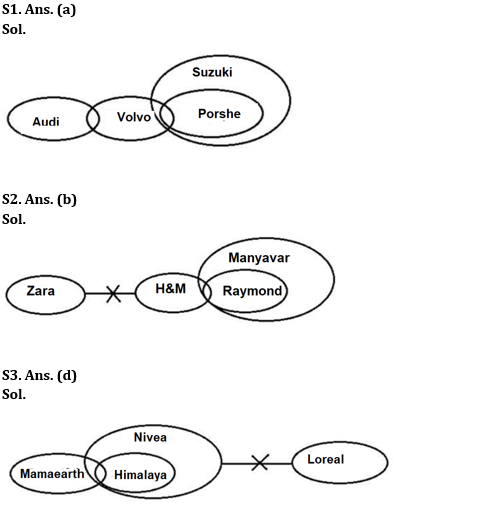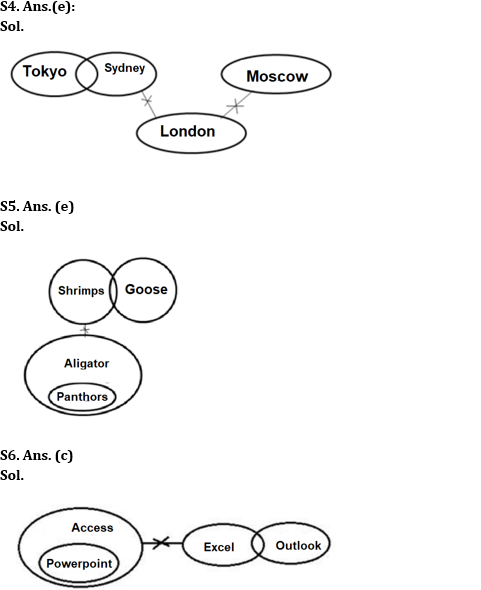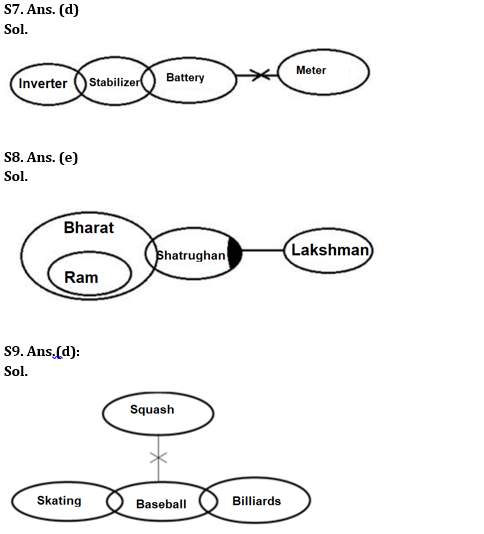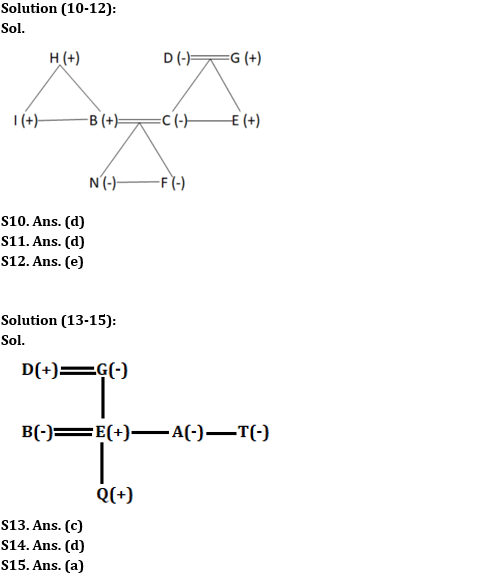Latest Banking jobs   »

# Reasoning Ability Quiz For IBPS RRB PO Prelims 2022- 14th June

Directions (1-4): In the question below, few statements are given followed by two conclusions numbered I, and II. You have to take the given statements to be true even if they seem to be at variance with commonly known facts. Read all the conclusions and then decide which of the given conclusions logically follows from the given statements disregarding commonly known facts.

Q1. Statements: Some Audi are Volvo. Only a few Volvo are Porshe. All Porshe are Suzuki.
Conclusions:
I. Some Audi being Porshe is a possibility.
II. Some Volvo are not Suzuki.

(a) If only conclusion I follows
(b) If only conclusion II follows
(c) If either conclusion I or conclusion II follows
(d) If both conclusions I and II follow
(e) If neither conclusion I nor conclusion II follows

Q2. Statements: No Zara is H&M. Only a few H&M are Raymond. All Raymond are Manyavar.
Conclusions:
I. No Zara is Raymond
II. Some Manyavar are not Zara

(a) If only conclusion I follows
(b) If only conclusion II follows
(c) If either conclusion I or conclusion II follows
(d) If both conclusions I and II follow
(e) If neither conclusion I nor conclusion II follows

Q3. Statements: Only a few Mamaearth are Himalaya. All Himalaya are Nivea. No Nivea are Loreal.
Conclusions:
I. Some Mamaearth can never be Loreal
II. No Himalaya is Loreal

(a) If only conclusion I follows
(b) If only conclusion II follows
(c) If either conclusion I or conclusion II follows
(d) If both conclusions I and II follow
(e) If neither conclusion I nor conclusion II follows

Q4. Statements: No London is Moscow. Only a few Tokyo are Sydney. No Sydney are London.
Conclusions:
I. Some Sydney is not Tokyo
II. Some Moscow is Tokyo

(a) If only conclusion I follows
(b) If only conclusion II follows
(c) If either conclusion I or conclusion II follows
(d) If both conclusions I and II follow
(e) If neither conclusion I nor conclusion II follows

Directions (5-9): In the question below, few statements are given followed by two conclusions numbered I, and II. You have to take the given statements to be true even if they seem to be at variance with commonly known facts. Read all the conclusions and then decide which of the given conclusions logically follows from the given statements disregarding commonly known facts.

Q5. Statements: All Panthers are Aligators. Only a few Goose are Shrimp. No Shrimp is Aligator.
Conclusions:
I. All Shrimps are Panthers
II. Some Gooses are Panthers

(a) If only conclusion I follows
(b) If only conclusion II follows
(c) If either conclusion I or conclusion II follows
(d) If both conclusions I and II follow
(e) If neither conclusion I nor conclusion II follows

Q6. Statements: All Powerpoints are Access. No Access is Excel. Only a few Excel is Outlook.
Conclusions:
I. Some Access is Outlook.
II. No Access is Outlook.

(a) If only conclusion I follows
(b) If only conclusion II follows
(c) If either conclusion I or conclusion II follows
(d) If both conclusions I and II follow
(e) If neither conclusion I nor conclusion II follows

Q7. Statements: Only a few Inverter is Stabilizer. Only a few Stabilizer is Battery. No Battery is Meter.
Conclusions:
I. Some Stabilizer is not Meter
II. Some Inverter is not Stabilizer

(a) If only conclusion I follows
(b) If only conclusion II follows
(c) If either conclusion I or conclusion II follows
(d) If both conclusions I and II follow
(e) If neither conclusion I nor conclusion II follows

Q8. Statements: All Ram are Bharat. Only a few Bharat are Shatrughan. Some Shatrughan are not Laxman.
Conclusions:
I. Some Bharat are not Laxman
II. No Ram is Shatrughan

(a) If only conclusion I follows
(b) If only conclusion II follows
(c) If either conclusion I or conclusion II follows
(d) If both conclusions I and II follow
(e) If neither conclusion I nor conclusion II follows

Q9. Statements: No Squash is Baseball. Some Skatings are Baseballs. Only a few Billiards are Baseballs.
Conclusions:
I. All Baseball being Billiards is a possibility.
II. Some Skating being Billiards is a possibility.

(a) If only conclusion I follows
(b) If only conclusion II follows
(c) If either conclusion I or conclusion II follows
(d) If both conclusions I and II follow
(e) If neither conclusion I nor conclusion II follows

Directions (10-12): Study the information carefully and answer the questions accordingly.
There are nine members in a family with four females and two married couples. B is the son-in-law of D. C is the daughter-in-law of H who is the father of I. N is the granddaughter of D. I is unmarried. E is the son of D. D is married to G who is the grandfather of F. F is the niece of I who is the brother of B. There could be single parent in the family.

Q10. Who is the mother of N?
(a) The daughter of H
(b) The wife of E
(c) F
(d) C
(e) None of these

Q11. How E is related to F?
(a) Grandfather
(b) Father
(c) Paternal uncle
(d) Maternal uncle
(e) None of these

Q12. Four from the following five are similar in a certain way and forms a group. Find out the one who does not belong to that group?
(a) I
(b) E
(c) B
(d) C
(e) F

Directions (13-15): Study the information carefully and answer the questions accordingly.
A three-generation family consists of seven members. B is daughter-in-law of D. G is spouse of D and mother of three children in which only one is male. T’s nephew is the child of E. E is only brother-in-law of A and T. Q is one of the members in the family.

Q13. How many male members are there in the family?
(a) Four
(b) Two
(c) Three
(d) Either four or three
(e) Can’t be determined

Q14. What is the relation of Q with respect to D?
(a) Sister
(b) Brother
(c) Son-in-law
(d) Grandson
(e) None of these

Q15. Who is the nephew of A?
(a) Q
(b) D
(c) Can’t be determined
(d) B
(e) Either Q or B

Solutions#### Congratulations!